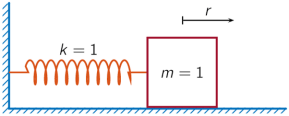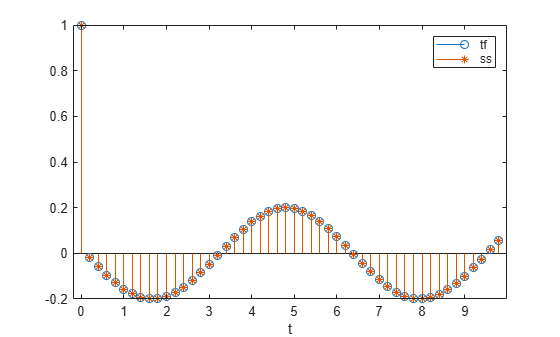tf2ss

Convert transfer function filter parameters to state-space form

Description

example

[A,B,C,D] = tf2ss(b,a) converts a continuous-time or discrete-time single-input transfer function into an equivalent state-space representation.

Examples

collapse all

Consider the system described by the transfer function

$H\left(s\right)=\frac{\left[\begin{array}{c}2s+3\\ {s}^{2}+2s+1\end{array}\right]}{{s}^{2}+0.4s+1}.$

Convert it to state-space form using tf2ss.

b = [0 2 3; 1 2 1];
a = [1 0.4 1];
[A,B,C,D] = tf2ss(b,a)
A = 2×2

-0.4000   -1.0000
1.0000         0

B = 2×1

1
0

C = 2×2

2.0000    3.0000
1.6000         0

D = 2×1

0
1

A one-dimensional discrete-time oscillating system consists of a unit mass, $\mathit{m}$, attached to a wall by a spring of unit elastic constant. A sensor samples the acceleration, $\mathit{a}$, of the mass at ${\mathit{F}}_{\mathrm{s}}=5$ Hz.Generate 50 time samples. Define the sampling interval $\Delta \mathit{t}=1/{\mathit{F}}_{\mathrm{s}}$.

Fs = 5;
dt = 1/Fs;
N = 50;
t = dt*(0:N-1);
u = [1 zeros(1,N-1)];

The transfer function of the system has an analytic expression:

$\mathit{H}\left(\mathit{z}\right)=\frac{1-{\mathit{z}}^{-1}\left(1+\mathrm{cos}\Delta \mathit{t}\right)+{\mathit{z}}^{-2}\mathrm{cos}\Delta \mathit{t}}{1-2{\mathit{z}}^{-1}\mathrm{cos}\Delta \mathit{t}+{\mathit{z}}^{-2}}$.

The system is excited with a unit impulse in the positive direction. Compute the time evolution of the system using the transfer function. Plot the response.

bf = [1 -(1+cos(dt)) cos(dt)];
af = [1 -2*cos(dt) 1];
yf = filter(bf,af,u);

stem(t,yf,'o')
xlabel('t')Find the state-space representation of the system. Compute the time evolution starting from an all-zero initial state. Compare it to the transfer function prediction.

[A,B,C,D] = tf2ss(bf,af);

x = [0;0];
for k = 1:N
y(k) = C*x + D*u(k);
x = A*x + B*u(k);
end

hold on
stem(t,y,'*')
hold off
legend('tf','ss')Input Arguments

collapse all

Transfer function numerator coefficients, specified as a vector or matrix. If b is a matrix, then each row of b corresponds to an output of the system.

• For discrete-time systems, b contains the coefficients in descending powers of z.

• For continuous-time systems, b contains the coefficients in descending powers of s.

For discrete-time systems, b must have a number of columns equal to the length of a. If the numbers differ, make them equal by padding zeros. You can use the function eqtflength to accomplish this.

Transfer function denominator coefficients, specified as a vector.

• For discrete-time systems, a contains the coefficients in descending powers of z.

• For continuous-time systems, a contains the coefficients in descending powers of s.

Output Arguments

collapse all

State matrix, returned as a matrix. If the system is described by n state variables, then A is n-by-n.

Data Types: single | double

Input-to-state matrix, returned as a matrix. If the system is described by n state variables, then B is n-by-1.

Data Types: single | double

State-to-output matrix, returned as a matrix. If the system has q outputs and is described by n state variables, then C is q-by-n.

Data Types: single | double

Feedthrough matrix, returned as a matrix. If the system has q outputs, then D is q-by-1.

Data Types: single | double

collapse all

Transfer Function

tf2ss converts the parameters of a transfer function representation of a given system to those of an equivalent state-space representation.

• For discrete-time systems, the state-space matrices relate the state vector x, the input u, and the output y:

$\begin{array}{c}x\left(k+1\right)=Ax\left(k\right)+Bu\left(k\right),\\ y\left(k\right)=Cx\left(k\right)+Du\left(k\right).\end{array}$

The transfer function is the Z-transform of the system’s impulse response. It can be expressed in terms of the state-space matrices as

$H\left(z\right)=C{\left(zI-A\right)}^{-1}B+D.$

• For continuous-time systems, the state-space matrices relate the state vector x, the input u, and the output y:

$\begin{array}{l}\stackrel{˙}{x}=Ax+Bu,\\ y=Cx+Du.\end{array}$

The transfer function is the Laplace transform of the system’s impulse response. It can be expressed in terms of the state-space matrices as

$H\left(s\right)=\frac{B\left(s\right)}{A\left(s\right)}=\frac{{b}_{1}{s}^{n-1}+\cdots +{b}_{n-1}s+{b}_{n}}{{a}_{1}{s}^{m-1}+\cdots +{a}_{m-1}s+{a}_{m}}=C{\left(sI-A\right)}^{-1}B+D.$